Let the 2-D cylinder function be defined by(1)

Then the Radon transform is given by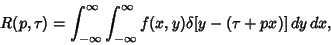(2)

where(3)

is the Delta Function.(4)
Now write(5)

witha phase shift. Then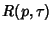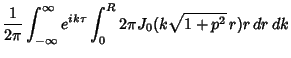(6)

Then use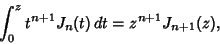(7)

which, with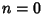, becomes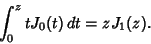(8)

Define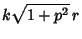(9)(10)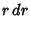(11)

so the inner integral is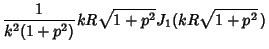(12)(13)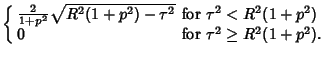(14)

Converting to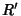using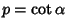,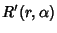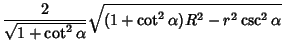(15)

which could have been derived more simply by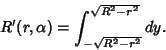(16)